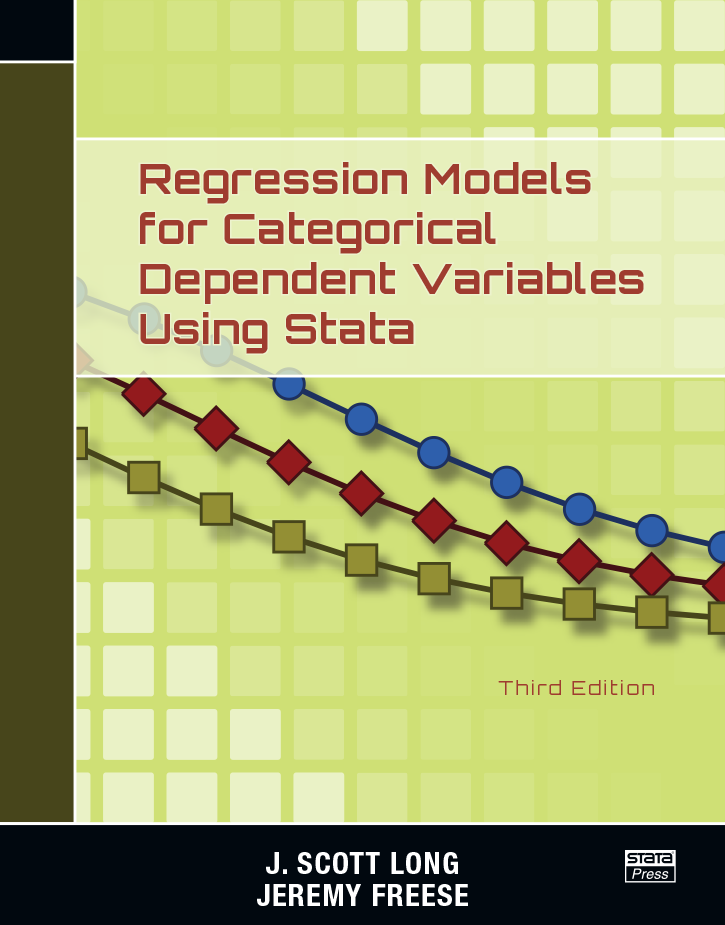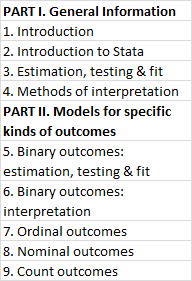## Monday, October 13, 2014

### Regression Models for Categorical Dependent Variables using Stata by J. Scott Long & Jeremy Freese (3rd edition)Scott Long and Jeremy Freese have released the third edition of their book "Regression Models for Categorical Dependent Variables using Stata", their first update since the 2nd edition came out in 2006 (here is a review of that edition by Richard Williams). Many thanks to Timberlake and Stata Press for sending the blog an advance copy. It is a beautiful piece of work which I will be using as my main Stata reference for the foreseeable future.

The new edition is a hefty 589 pages, a significant increase from the 311 pages in the 2nd edition. The book focuses on categorical outcome variablesor outcomes with two or more possible values. These kind of outcomes require non-linear models to properly analyze, such as Probit, Logit, negative binomial or Multinominal Probit/Logit, rather than the basic OLS which is used for linear models. When dealing with non-linear models, "the simple interpretations that are possible in linear models are [not] appropriate... Because of this nonlinearity, no method of interpretation can fully describe the relationships among the independent variables and the outcomes. Rather, a series of postestimation explorations are needed to uncover the most important aspects of these relationships. If you limit your interpretations to the standard output of estimated slope coefficients, your interpretation will usually be incomplete and sometimes even misleading" [p7].

The book's index is shown in Fig. 1 and described further here.Fig 1. Index
Part I begins with a concise introduction to Stata and a review of the fundamentals of model estimation, making the book relatively accessible for researchers unfamiliar with Stata. Part II describes how to estimate and interpret binary, ordinal, nominal, and count outcomes. Throughout the text the authors use clear language and many practical examples to emphasize the intuition behind the various analytic techniques without a lot of dense mathematics.

Although the book is a good introduction to Stata, the real added value comes from the enormous level of detail the authors devote to describing how to interpret (and graph) regression results using the margins command and a suite of supplemental post-estimation commands created by the authors called mgen, mchange and mtable (these latter three commands are collectively called SPost13 and replace the popular earlier suite SPost 9; see here for an explanation of why the authors recommend using SPost13 rather than margins). Chapter 4 is devoted entirely to describing these post-estimation commands and chapters 5-9 contain many example applications of them for different kinds of models. The authors also provide free example data-sets and code to practise these commands on, which can be downloaded by following the instructions in the book [p13].

The authors highlight that margins excels at four things in particular [p137], all of which are described in further detail here. These are:
(1) Predictions for each observation
Margins can predict the probability of an outcome for each person in the data, taking into account all the covariates included in the regression. The predict command can do this, but margins also provides standard errors and confidence intervals.
(2) Predictions at specified values
Margins can compute the probability of an outcome at specific values of covariates while holding the others constant or at their means (or at any other value).
(3) Marginal effects
Margins can compute how changes in a covariate are associated with changes in an outcome variable, holding other covariates constant.
(4) Graphs of predictions
Marginsplot can easily graph outcome variables based on margins. Commands such as marginsplot and Ben Jann's coefplot are particularly good at turning potentially obtuse interaction coefficients into intuitive graphs such as (i) age*age (ii) gender*race (see p43) (iii) age*race (see p24-25).

This book is ideal for graduate students (like myself), who may wish to work their way through Chapters 1-4 in detail. More experienced researchers could start with Chapters 4-6, which really unpack the margins command and methods of testing and interpreting non-linear coefficients. Although the title of the book focuses on categorical dependent variables, there is plenty for researchers working with continuous outcomes to learn from it given how useful the margins and SPost13 commands are for clarifying all manner of results.

I'll be using this book as a reference for a series of posts over the next few months which further explore the capabilities of margins, SPost13 and marginsplot. These will be available under the 'Stata resources' tab on the left hand side.

 Categorical variables are distinct from continuous variables because they don't have any intrinsic ordering. Examples include (0 = employed, 1 = unemployed, 2 = in education) or (0 = smoker, 1 = not a smoker). An example of a continuous variable is a measure of intelligence where the scores range from low to high along a common scale (i.e. 1-100).
 Binary outcomes have two values such as whether a person is healthy or sick. Ordinal outcomes have more than two categories that are assumed to be ordered on a single, underlying dimension such as the answers to a survey question ranging from "Strongly disagree" -  "Disagree" - "Neither agree nor disagree" - "Agree" - "Strongly agree". Nominal outcomes have more than two categories but the categories are not ordered, such as whether a person travels to work by car, train, bus or foot. Count variables count the number of times something has happened, such as the number of months a person has been unemployed for, or the number of articles written by a scientist [all definitions taken from p8].
 Until now, my own knowledge of the margins command has been gleaned from online guides and presentations, so it is nice to finally have an authoritative text to refer to.Anonymous said...

any suggestions of equivalent texts for R users?Anonymous said...

An OLS regression takes the form
y= β0 + Xβ1 + Xβ2 + Xβ3 + ε

What form does an Ordered Logistic Regression takes?

How can β0 be obtained from the ologit regression output in STATA.

Thanks